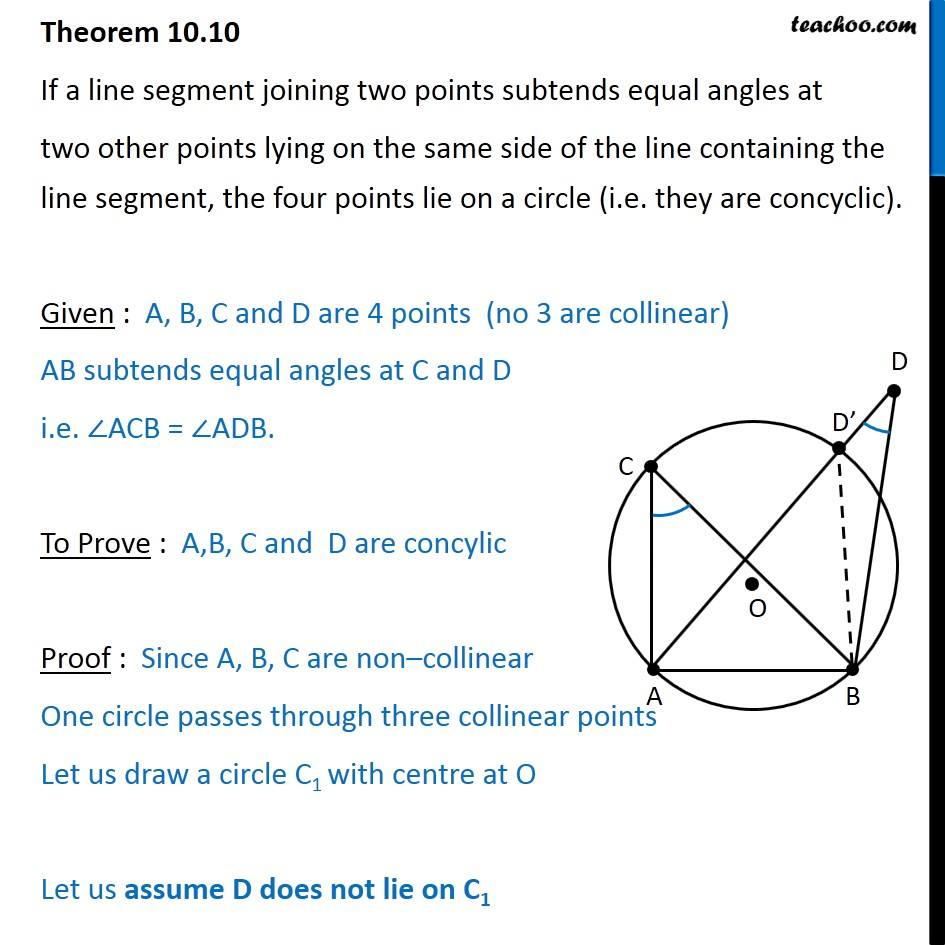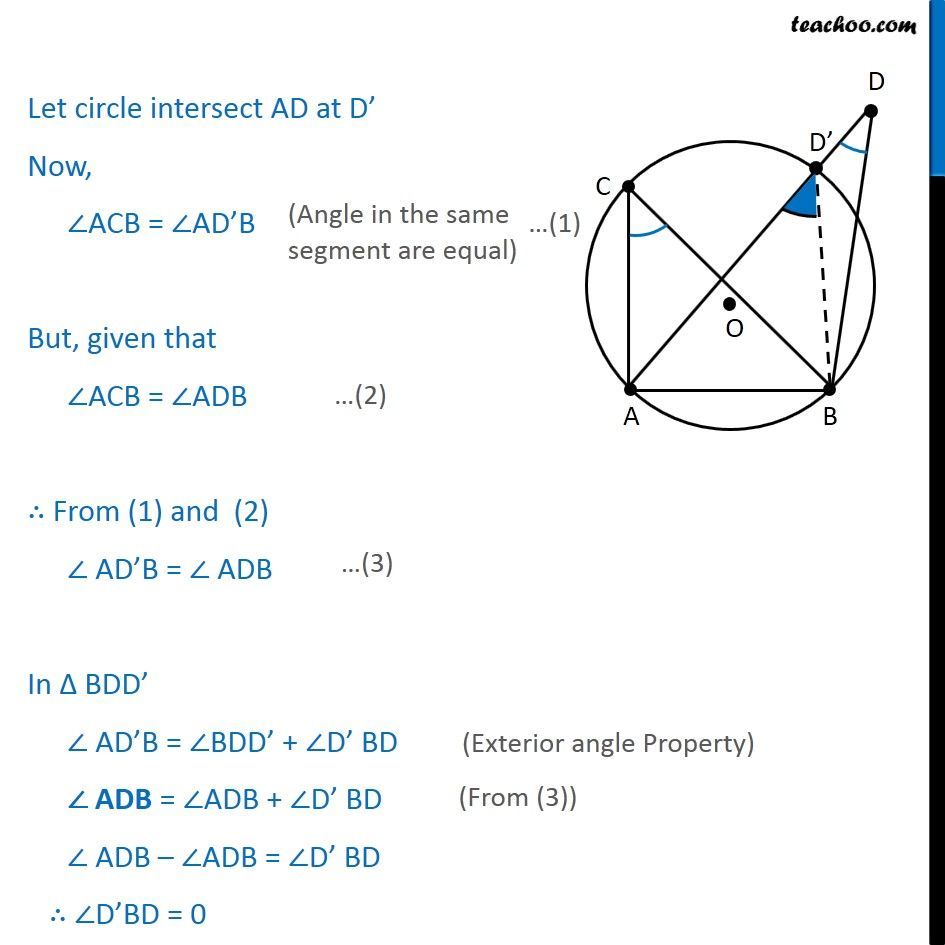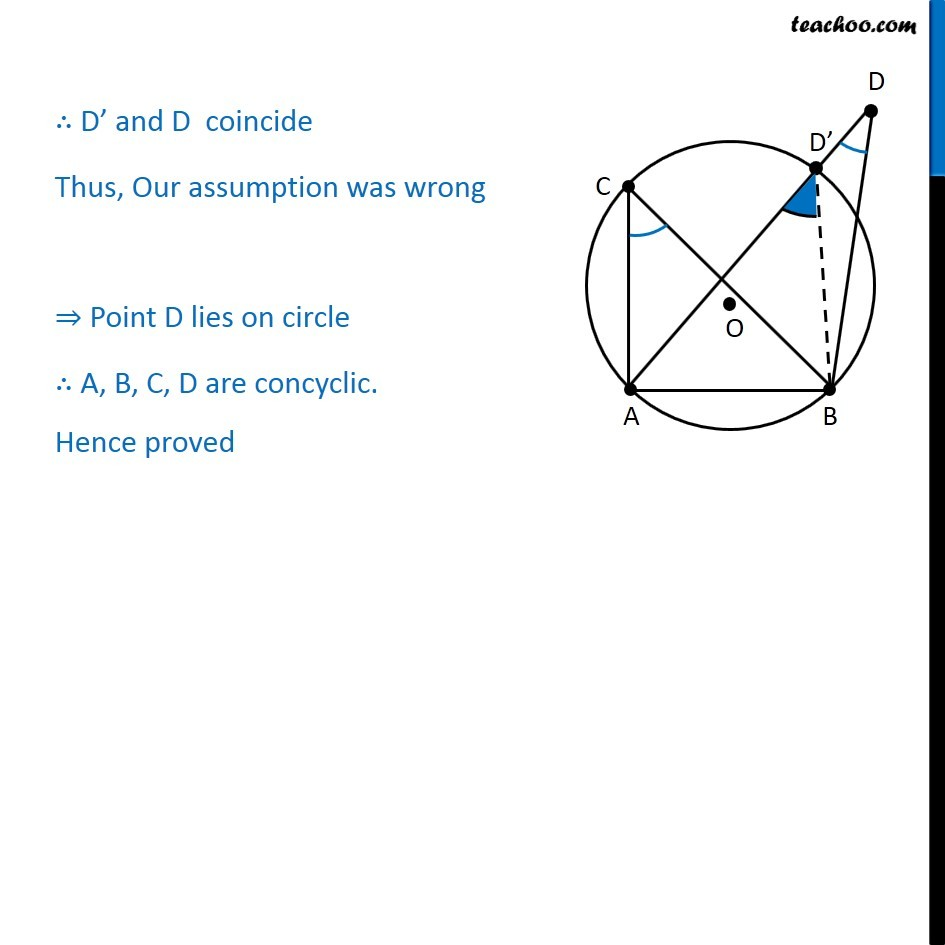1. Chapter 10 Class 9 Circles (Term 2)
2. Serial order wise
3. Theorems

Transcript

Theorem 10.10 If a line segment joining two points subtends equal angles at two other points lying on the same side of the line containing the line segment, the four points lie on a circle (i.e. they are concyclic). Given : A, B, C and D are 4 points (no 3 are collinear) AB subtends equal angles at C and D i.e. ∠ACB = ∠ADB. To Prove : A,B, C and D are concylic Proof : Since A, B, C are non–collinear One circle passes through three collinear points Let us draw a circle C1 with centre at O Let us assume D does not lie on C1 Let circle intersect AD at D’ Now, ∠ACB = ∠AD’B But, given that ∠ACB = ∠ADB ∴ From (1) and (2) ∠ AD’B = ∠ ADB In ∆ BDD’ ∠ AD’B = ∠BDD’ + ∠D’ BD ∠ ADB = ∠ADB + ∠D’ BD ∠ ADB – ∠ADB = ∠D’ BD ∴ ∠D’BD = 0 ∴ D’ and D coincide Thus, Our assumption was wrong ⇒ Point D lies on circle ∴ A, B, C, D are concyclic. Hence proved

Theorems